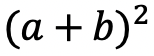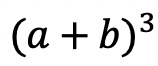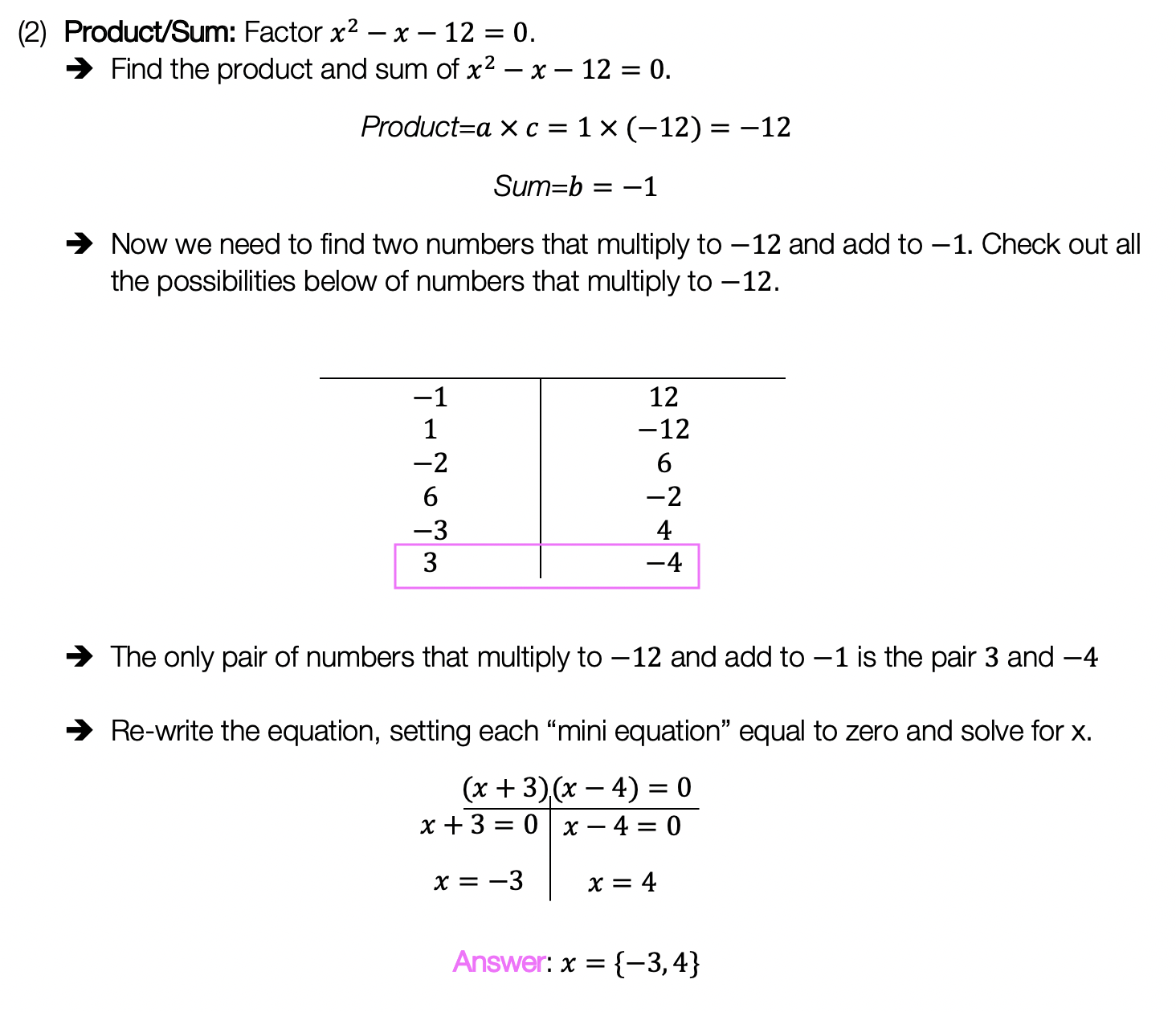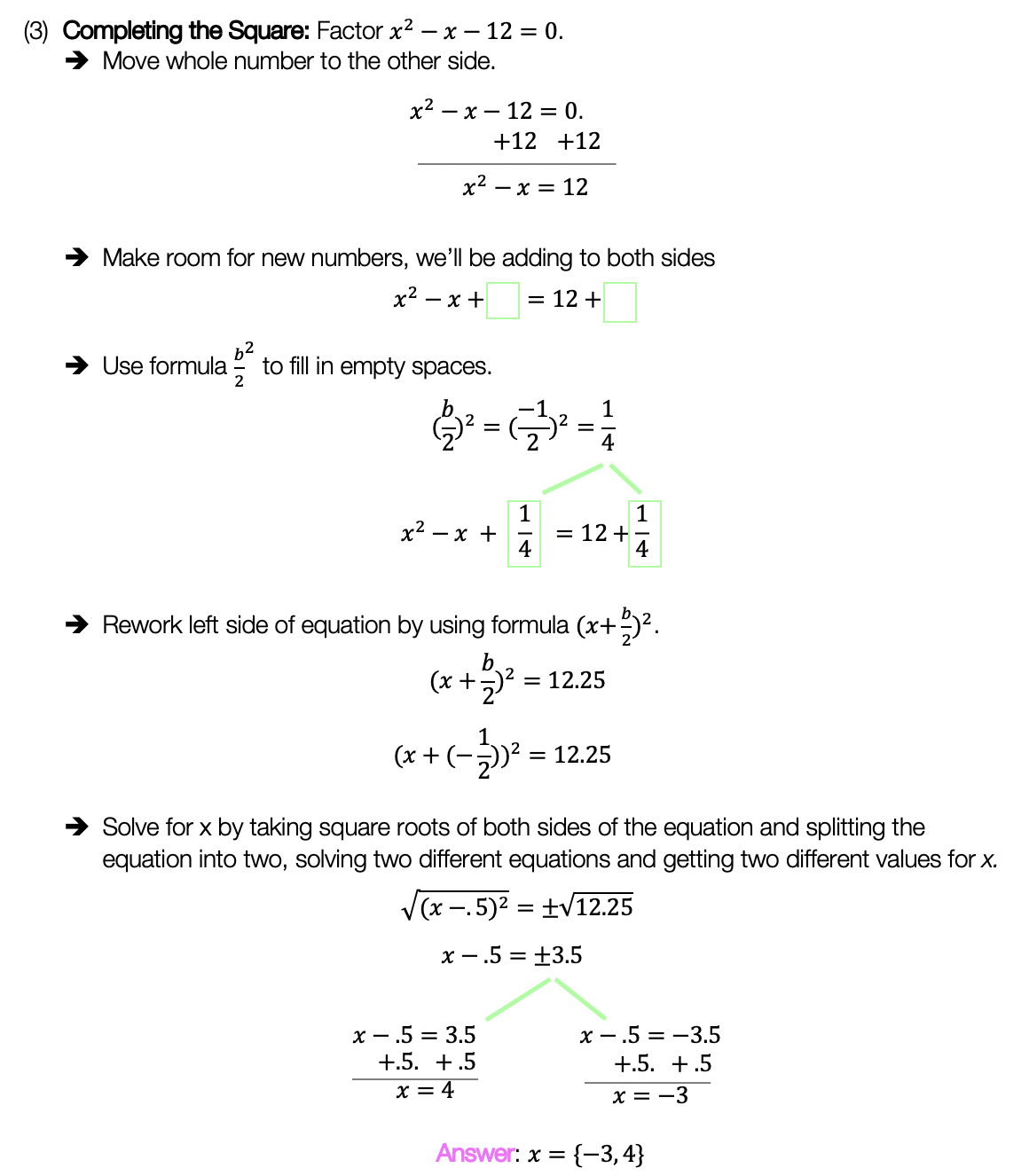## Infinite Geometric Series Formula

Hey math friends and happy Wednesday! Today we are going to take a look at the infinite geometric series formula in summation notation. First, we’ll review what a geometric sequence (or geometric progression) is, then look at the difference between divergence and convergence based on the common ratio, and then finally look at the formula. The unique thing about infinite geometric sequences is that the formula doe snot always apply. We only know if the formula applies to our series based on the value of the common ratio.

This may sound a bit complicated, with a few new concepts, but fear not as divergence and convergence are easy to identify! If you have any questions, don’t forget to check out the video and practice problems below. Happy calculating! 🙂

## What does it mean to find the “Sum of Infinite Geometric Sequence” (Series)?

We already know what a geometric sequence is: a sequence of numbers that forms a pattern when the same number is multiplied or divided by each successive term.

The number is multiplied or divided to each term is called the common ratio. We can see below that each number in the sequence is being multiplied by 1/2. Therefore, we can say that the common ratio of the geometric sequence below is 1/2.

Example:

But then, what happens if we wanted to sum all the terms of our geometric sequence together? The sequence of terms is now considered to be an infinite geometric series since we are summing all of the terms of our series together, infinitely. Take a look below:

Example:

## Summation Notation:

We can also write our infinite geometric series using something called Summation Notation shown below. This is a different format, also known as sigma notation, if you need a refresher on how to use and read summation notation, please check out the link in this paragraph. When writing in summation notation, we are actually writing the sum of the geometric series in a different way. Check it out below:

Now that we know what each part of this summation notation looks like, let’s actually identify what each part of this equation means in relation to our geometric sequence:

a1=The first term. In this case, we can see that the first term of our geometric sequence is the number 5 in the example below. Therefore, we can say a1=5.

r= The common ratio is the number that is multiplied or divided by each consecutive term within the sequence. In the example below, each number is multiplied by 1/2, therefore we can say, r=1/2.

n= The total number of terms we are trying to sum together. In the example mentioned below, we are trying to sum an infinite number of terms, so in this case, n starts at 1 and then continues infinitely to increase by 1 for each subsequent term.

If we were to expand our inifite series summation it would look something like this:

But how would we calculate this? This is an infinite series represented in summation notation, therefore, it goes on infinitely, how does this work?

That’s where our Infinite Geometric Series formula comes in handy! But before we jump into finding a solution and looking at our formula, let us take a closer look at what types of geometric series qualify for using the infinite series formula in the first place.

## Divergence Vs. Convergence: When do we use the Infinite Series formula?

There are two types of Infinite Geometric Series:

Type 1: Infinite Geometric Series that diverges to infinity

Type 2: Infinite Geometric Series that converges to a numeric value (-1 < r < 1)

Check out the differences in the example below:

## Infinite Geometric Series Formula:

Now the moment you’ve all been waiting for… the Inifite Geometric Series Formula! Remember, the use of this formula is purely based on the common ratio of our geometric sequence.

The infinite geometric series formula can only be used if the common ratio, r, is less than 1 and greater than -1. If the common ratio, r, is not between -1 and 1, then the sum of the geometric sequence diverges to infinity (and the formula cannot be used).

Now that we have the formula to work with and know when to use it (when -1 < r < 1), let’s take another look at our question and apply our infinite geometric series formula to find a solution:

Step 1: First, let’s identify the common ratio to make sure that it is between numbers -1 and 1. If the common ratio is a fraction between these two numbers, then we can use our infinite series formula.

Step 2: Now that we know we can use our infinite series formula, let’s write out each part of the formula and identify what numbers we are going to plug in.

Step 3: Last but not least, let’s fill in our formula with the correct numbers and solve with the given values.

Think you are ready to try inifite geometric series examples on your own? Check out the following infinite geometric series practice questions below to determine if a series converges or diverges.

## Practice Questions:

State if each given series converges or diverges, then if applicable find the solution.

## Related Posts:

Looking to learn more about sequences? You’ve come to the right place! Check out these sequence resources and posts below. Personally, I recommend looking at the finite series posts next including: finite geometric sequence or finite arithmetic series!

Arithmetic Sequence (arithmetic progression)

Recursive Formula

Finite Arithmetic Series

Finite Geometric Series

Infinite Geometric Series

Golden Ratio in the Real World

Fibonacci Sequence

Still, got questions? No problem! Don’t hesitate to comment below or reach out via email. And if you would like to see more MathSux content, please help support us by following ad subscribing to one of our platforms. Thanks so much for stopping by and happy calculating!

## Transforming Trig Functions: Amplitude, Frequency, Period, Phase Shifts

Hi everyone, and welcome to MathSux! In this post, we are going to break down transforming trig functions by identifying their amplitude, frequency, period, horizontal phase shift, and vertical phase shifts. Fear not! Because we will break down what each of these terms (amplitude, frequency, period, horizontal phase shift and vertical phase shifts) mean and how to find them when looking at a trigonometric function and then apply each of these changes step by step to our graph. In this particular post, we will be transforming and focusing on a cosine function, but keep in mind that the same rules apply for transforming sine functions as well (example shown below in practice).

And if you’re ready for more, check out the video and the practice problems below, happy calculating! 🙂

*For a review on how to derive the basic Trig functions (y=sinx, y=cosx, and y=tanx), click here.

## What are the Different Parts of a Trig Function?

When transforming trig functions, there are several things to look out for, let’s take a look at what each part of a trig function represents below:

Amplitude: The distance (or absolute value) between the x axis and the highest point on the graph.

Frequency: This is the number of cycles that happen between 0 and 2π. (Α “cycle” in this case is the number of “s” cycles for the sine function).

Period: The x-value/length of one cycle. (Α “cycle” in this case is the number of “s” cycles for the sine function). This is found by looking at the graph and seeing where the first cycle ends, or, by using the formula:

Horizontal Shift: When a trigonometric function is moved either left or right along the x axis.

Vertical Shift: When a trigonometric function is moved either up or down along the y axis.

Let’s try an Example, by graphing the following trig function step by step by identifying the amplitude, frequency, period, vertical shift, and horizontal phase shift.

Step 1: First let’s label and identify all the different parts of our trig function and what each part represents.

Step 2: Now let’s transform our trig graph one step at a time.  First, let’s start graphing y=cos(x) without any transformations, the basic graph.

Step 3: Next, let’s add our amplitude of 2, otherwise known as the height, or distance to the x-axis.  To do this our highest and lowest points on the y-axis will now be moved to 2 and -2 respectively.

Step 4: Next, we can apply our horizontal shift to the left by (π/2) or 90º.  To do this, we need to look at where negative (π/2) is on our graph at (-π/2) and move our entire graph over to start at this new point, “shifting” over each coordinate point by (π/2) along the x axis.

Step 5: For our last transformation, we have a vertical phase shift up 1 unit.  All this means is that we are going to shift our entire graph up by 1 unit along the y axis.

Think you are ready to try graphing trig functions and identifying the amplitude, frequency, period, vertical phase shift, and horizontal phase shift? Check out the practice questions and answers below!

## Practice Questions:

When you’re ready check out the function transformations solutions below:

## Solutions:

Still, got questions? No problem! Don’t hesitate to comment with any questions or check out the video above for an in-depth explanation. Happy calculating! 🙂

## Related Trigonometry Posts:

The Unit Circle

Basic Right Triangle Trigonometric Ratios (SOH CAH TOA)

4545 90 Special Triangles

30 60 90 Special Triangles

Graphing Trigonometric Functions

Trig Identities

Factoring Trigonometric Functions

Law of Cosines

Law of Sines

## Synthetic Division and Factoring Polynomials: Algebra 2/Trig.

Hey there math friends! In this post we will go over how and when to use synthetic division to factor polynomials! So far, in algebra we have gotten used to factoring polynomials with variables raised to the second power, but this post explores how to factor polynomials with variables raised to the third degree and beyond!

If you have any questions don’t hesitate to comment or check out the video below. Also, don’t forget to master your skills with the practice questions at the end of this post. Happy calculating! 🙂

## What is Synthetic Division?

Synthetic Division is a shortcut that allows us to easily divide polynomials as opposed to using the long division method. We can only use synthetic division when we divide a polynomial by a binomial in the form of (x-c), where c is a constant number.

## Example #1:

*Notice we can use synthetic division in this case because we are dividing by (x+4) which follows our parameters (x-c), where c is equal to 4.

## Example #2: Factoring Polynomials

Let’s take a look at the following example and use synthetic division to factor the given polynomial:

Check!

The great thing about these questions is that we can always check our work! If we wanted to check our answer, we could simply distribute 2(x+1)(x+3)(x-2) and get our original polynomial, f(x)=2x3+4x2-10x-12.

Try the practice problems on your own below!

Looking to brush up on how to divide polynomials the long way using long division? Check out this post here!

Still got questions? No problem! Don’t hesitate to comment with any questions or check out the video above. Happy calculating! 🙂

## Expanding Cubed Binomials: Algebra 2/Trig.

Greetings math friends! This post will go over expanding cubed binomials using two different methods to get the same answer. We’re so used to seeing squared binomials such as,, and expanding them without a second thought.  But what happens when our reliable squared binomials are now raised to the third power, such as,?  Luckily for us, there is a Rule we can use:

But where did this rule come from?  And how can we so blindly trust it? In this post we will prove why the above rule works for expanding cubed binomials using 2 different methods:

Why bother? Proving this rule will allow us to expand and simplify any cubic binomial given to us in the future! And since we are proving it 2 different ways, you can choose the method that best works for you.

## Method #1: The Box Method

Step 1: First, focus on the left side of the equation by expanding (a+b)3:

Step 2: Now we are going to create our first box, multiplying (a+b)(a+b). Notice we put each term of (a+b) on either side of the box. Then multiplied each term where they meet.

Step 3: Combine like terms ab and ab, then add each term together to get a2+2ab+b2.

Step 4: Multiply (a2+2ab+b2)(a+b) making a bigger box to include each term.

Step 5: Now combine like terms (2a2b and a2b) and (2ab2 and ab2), then add each term together and get our answer: a3+3a2b+3ab2+b3.

## Method #2: The Distribution Method

Let’s expand the cubed binomial using the distribution method step by step below:

Now that we’ve gone over 2 different methods of cubic binomial expansion, try the following practice questions on your own using your favorite method!

Practice Questions: Expand and simplify the following.

Solutions:

Still, got questions?  No problem! Check out the video above or comment below! Happy calculating! 🙂

**Bonus: Test your skills with this Regents question on!

## 4 Ways to Factor Trinomials: Algebra

Greeting math peeps and welcome to MathSux! In this post, we are going to go over 4 ways to Factor Trinomials and get the same answer, including, (1) Quadratic Formula (2) Product/Sum, (3) Completing the Square, and (4) Graphing on a Calculator.  If you’re looking for more don’t forget to check out the video and practice questions below.  Happy Calculating! 🙂

Also, if need a review on Factor by Grouping or Difference of Two Squares (DOTS) check out the hyperlinks here!

*If you haven’t done so, check out the video that goes over this exact problem, and don’t forget to subscribe!

We will take this step by step, showing 4 ways to factor trinomials, getting the same answer each and every time! Let’s get to it!

## 4 Ways to Factor Trinomials

____________________________________________________________________

## (2) Product/Sum:____________________________________________________________________

## (3) Completing the Square:____________________________________________________________________

## (4) Graph:

Choose the factoring method that works best for you and try the practice problems on your own below!

Practice Questions:

Solutions:

Want a review of all the different factoring methods out there?  Check out the ones left out here (DOTS and GCF) and happy calculating! 🙂

For even more ways to factor quadratic equations, check out How to factor by Grouping here! 🙂

Looking for more on Quadratic Equations and functions? Check out the following Related posts!

Factoring Review

Factor by Grouping

Completing the Square

The Discriminant

Is it a Function?

Imaginary and Complex Numbers

Quadratic Equations with 2 Imaginary Solutions

Focus and Directrix of a Parabola

Also, if you want more Mathsux?  Don’t forget to check out our Youtube channel and more below! If you have any questions, please don’t hesitate to comment below. Happy Calculating! 🙂